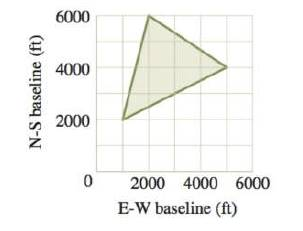Chapter 11.4, Problem 73E### Algebra and Trigonometry (MindTap ...

4th Edition
James Stewart + 2 others
ISBN: 9781305071742

#### Solutions

Chapter
Section### Algebra and Trigonometry (MindTap ...

4th Edition
James Stewart + 2 others
ISBN: 9781305071742
Textbook Problem

# A Triangular Plot of Land An outdoors club is purchasing land to set up a conservation area. The last remaining piece they need to buy is the triangular plot shown in the figure. Use the determinant formula for the area of the triangle to find the area of the plot.To determine

To find:

The area of the plot using the determinant formula for the area of a triangle.

Explanation

Given:

An outdoors club is purchasing land to set up a conservation area. The last remaining piece they need to buy is the triangular plot shown in the figure.

Figure (1)

Approach:

The determinant is a value assigned to a square matrix.

The area of a triangle with vertices (a1,b1), (a2,b2) and (a3,b3) is given as,

A=±12|a1b11a2b21a3b31|(1)

Calculations:

Consider the figure of the plot.

From the figure, the coordinates of the triangle are (1000,2000), (2000,6000) and (5000,4000).

The area of the triangle is calculated using the equation (1)

### Still sussing out bartleby?

Check out a sample textbook solution.

See a sample solution

#### The Solution to Your Study Problems

Bartleby provides explanations to thousands of textbook problems written by our experts, many with advanced degrees!

Get Started